• pandas常用函数分类汇总，含有注释，分类pandas常用函数分类汇总
• new_series = df.groupby(by=['a'])['b'].sum() # 意思是对字段a进行分组然后通过字段B进行求和汇总 # 返回Series类型对象。 a会变成index b则成为值
new_series = df.groupby(by=['a'])['b'].sum()

# 意思是对字段a进行分组然后通过字段B进行求和汇总
# 返回Series类型对象。 a会变成index b则成为值


展开全文• >1)] #进行赛选 第三步，按条件进行分类汇总（按条件进行求和），进行按条件进行计数。 这里主要根据自己工作业务逻辑去做求和 和 计数 等计算方式 代码如下： province = sel_excel.groupby(['大区']).agg({'订单...

本人是在一家零售行业工作，所出的教程，都是我日常工作中遇到的 复杂而大量重复的工作 我用python代替去完成它，都是原创内容，非粘贴复制，如果我的文章能够帮助到大家，希望帮忙点个关注。当然，如果有很多错别字，也请见谅。

问题来了：
领导总是叫我每天导出来分析，但是我们公司分析数据总是要到 大区和小区，因为我们大区和小区都有负责人，KPI考核要对应负责人，通过数据去体现问题。这一次领导叫我把线上的门店自提订单数据导出来做数据分析。
题外话：因为公司对接到第三方ERP系统都是以门店为主去对接的，因为我们钱给的少，所以第三方数据不支持定制化数据分析。只能靠我们手工用excel 去做表。但是如果只是做一次，还勉强用excel 能做好。如果是天天都需要数据跟进，那天天都需要做数据赛选和匹配。虽然excel 可以做到输入好公式，把数据源复制粘贴进去，可以自动计算。但是excel 公式多，会非常的吃电脑性能，而且出现无响应等。所以这个时候我们需要利用代码去完成这个，我们叫的高大上的名字 办公自动化
先给大家看下从ERP导出来的数据源是什么样子：
订单状态订单金额（实付）下单时间提货门店销售门店（code）客服备注商品金额总计订单支付已过期0.102019-09-17 10:45:56多多一上总部A9999 0.10订单支付已过期0.102019-09-17 17:33:07江南摩尔店A9999 0.10订单支付已过期0.102019-09-17 17:33:59多多一上总部A9999 0.10订单支付已过期0.102019-09-17 17:35:04江南摩尔店A9999 0.10订单支付已过期0.102019-09-18 08:24:09江南摩尔店A9999 0.10订单支付已过期0.102019-09-18 08:28:07江南摩尔店A9999 0.10订单支付已过期0.102019-09-18 08:29:25嘉兴平湖店A9999 0.10订单支付已过期0.102019-09-18 08:53:05江南摩尔店A9999 0.10订单支付已过期0.102019-09-18 09:51:24多多一上总部A9999 0.10订单支付已过期0.102019-09-18 09:56:04多多一上总部A9999 0.10订单支付已过期0.102019-09-18 10:17:22多多一上总部A9999 0.10订单取消0.012019-09-18 10:55:52江南摩尔店A9999 0.01待备货29.002019-09-23 19:36:06兰溪星辰店A0012 29.00待备货29.002019-09-23 19:38:32巫山祥云店B0203 29.00订单支付已过期19.002019-09-23 19:38:51全椒新华路A0466 19.00订单取消29.002019-09-23 19:49:06宣恩解放街A0465 29.00订单支付已过期29.002019-09-23 19:49:12临沂兰山店A0265 29.00待备货29.002019-09-23 19:49:34枣庄薛城财富步行街A0295 29.00待备货67.002019-09-23 19:50:12三水湾店A0105 67.00待备货67.002019-09-23 19:50:12三水湾店A0105 67.00待备货67.002019-09-23 19:50:12三水湾店A0105 67.00
在数据合并前需要准备一个带有大区，小区 ，门店 的表去根据 “提货门店” 和 下方 的 “门店” 合并 如下表：我看到数据后先做的是 python 的 merge 数据合并，这里合并就是excel 函数的vlookup 是一样的基本功能，如果要区别的，我觉得merge 还是要强大。
大区小区门店南方大区温州大区温州楠江店南方大区温州大区温州新桥店南方大区温州大区温州状元店南方大区温州大区温州浦西店南方大区温州大区温州北白象新店南方大区温州大区路桥金清店南方大区温州大区温岭松门店南方大区温州大区温州蟠凤商业街南方大区温州大区区域合计北方大区江苏一区淮安幸福店北方大区江苏一区漕运广场店北方大区江苏一区东长街店北方大区江苏一区淮安盱眙店北方大区江苏一区阜宁阜师路北方大区江苏一区淮安汇通店北方大区江苏一区东大街店北方大区江苏一区少年宫店
代码如下：
#encoding:utf-8
import pandas as pd #导入pandas包
merge_excel = pd.merge(rd_excel1,rd_excel2,left_on='门店',right_on='提货门店') # 进行指定相同值的合并
merge_excel.to_excel(r"D:\111\merge.xlsx") #保存为merge.xlsx

合并后效果如下：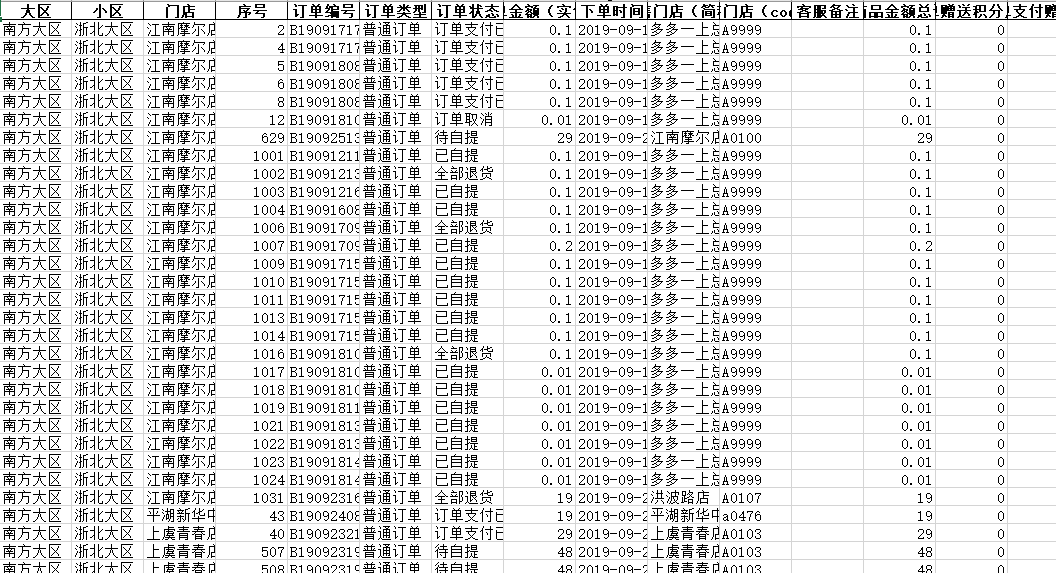事情还没完，这个只是在数据源中匹配合并好了提货门店所属的大区和小区
下面我们据需要根据上面的数据源 进行 对数据的分类汇总 和 计数 以及修改列名。
第一步，修改列名：
为了生成的数据更好理解 所以需要修改列名 。把数据源列名的 订单状态 修改为 订单数量，购买数量 修改为 购买件数
代码如下：
rd_merge = pd.read_excel(r"D:\111\merge.xlsx") #读取刚刚合并好的excel
changer =rd_merge.rename(columns={'订单状态':'订单数量','购买数量':'购买件数'}) #修改列名
第二步，赛选出不要的数值，这里是在excel 中 订单状态 那列除了“已自提”，“待备货”，“待自提” 且 商品总金额  大于0。
这里主要去除掉不要的数据，方便在生成的时候，不占用太多资源。
代码如下：
df = pd.DataFrame(changer) #讲上面改变列名的表格转成 DF
title = ['已自提','待备货','待自提'] #定义一个列表，就是需要选中的列的值
sel_excel = df[(df['订单数量'].isin(title)) & (df["商品金额总计"]>1)] #进行赛选

第三步，按条件进行分类汇总（按条件进行求和），进行按条件进行计数。
这里主要根据自己工作业务逻辑去做求和 和 计数 等计算方式
代码如下：
province = sel_excel.groupby(['大区']).agg({'订单数量':'count','商品金额总计':'sum','购买件数':'sum'})
to_city = sel_excel.groupby(['小区']).agg({'订单数量':'count','商品金额总计':'sum','购买件数':'sum'})
shop = sel_excel.groupby(['门店']).agg({'订单数量':'count','商品金额总计':'sum','购买件数':'sum'})

上述代码解释，以一行代码为例解释，
province = sel_excel.groupby(['大区']).agg({'订单数量':'count','商品金额总计':'sum','购买件数':'sum'})
按照上面“进行赛选” 后得出的数据进行 分组统计，也就是 groupby函数，然后集合agg函数，网上说agg 功能主要是提供基于列的聚合操作。在 groupby（）中的值 就是要计算的条件。而后面的出 在订单数量【前名字为订单状态】为计数，商品金额总计为求和，购买件数 为 求和 都是计算方式。

第四步，新建表格，将上面 大区，小区，门店，写入到excel 不同sheet中。
代码如下：
writer = pd.ExcelWriter(r"D:\111\m2.xlsx") #新建 m2.xlsx 表
sheet1 = pd.DataFrame(province) #进行转成DF格式
sheet2 = pd.DataFrame(to_city) #进行转成DF格式
sheet3 = pd.DataFrame(shop) #进行转成DF格式

sheet1.to_excel(writer,sheet_name='大区') #写入到m2.xlsx 的名叫 大区的sheet中
sheet2.to_excel(writer,sheet_name='小区') #写入到m2.xlsx 的名叫 小区的sheet中
sheet3.to_excel(writer,sheet_name='门店') #写入到m2.xlsx 的名叫 门店的sheet中
writer.save() #保存
writer.close() #关闭excel
最后代码执行后效果如下：
下方是sheet的截图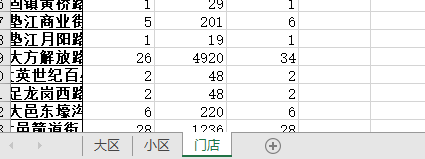下方是门店sheet中的数据：

门店订单数量商品金额总计购买件数万州五桥上海大道3873万州新城一店4964万州新城二店21162三台老西街2582三水湾店52495上虞青春店41924东大街店3773东长街店1043111中江上南街店2382临沂义堂店31453
下方是小区sheet中的数据：
小区订单数量商品金额总计购买件数安徽一区1850818安徽二区1966919安徽四区2886628山东一区54212556山东二区40187046江苏一区55191856江苏三区29121532浙北大区2496925浙西一区61826浙西二区1044810温州大区1793917
下方是大区sheet中的数据：
大区订单数量商品金额总计购买件数北方大区2439171255南方大区57253858成都大区39915550411湖南大区1123848116贵州大区1047487115重庆大区1244419126
分享就到这里了，可能文字描述逻辑有些不对，还请原谅。
别忘记，如果对你带来启发和灵感，给我点个关注呗。

展开全文• 有一批数据需要分类汇总和总计，看了一下pandas的groupby，可以实现。具体思路：先分组，分组后计算改分类的汇总小计，然后对dataframe进行拼接；分类汇总计算好了之后，计算总体的汇总，然后在进行拼接 具体代码： ...
有一批数据需要分类汇总和总计，看了一下pandas的groupby，可以实现。具体思路：先分组，分组后计算改分类的汇总小计，然后对dataframe进行拼接；分类汇总计算好了之后，计算总体的汇总，然后在进行拼接
具体代码：
"""
pandas 实现分类汇总 总计
"""
import os
import sys

BASE_DIR = os.path.dirname(os.path.dirname(os.path.abspath(__file__)))

sys.path.append(BASE_DIR)

import pandas as pd

def gen_pivot_table(df, calculate_fields, special_fields, groupby_fields):
"""
:param df:
:param calculate_fields: 需要计算的字段
:param special_fields: 需要进行特殊计算的字段  两列比值 两列之和/差 等
:param groupby_fields: 需要分组的字段
:return:
"""
last_total_df = calculation_total(df, calculate_fields, special_fields, name=u"总计")

print("last_total_df: {}\n".format(last_total_df))

sub_total_df = calculation_sub_total(df, groupby_fields, calculate_fields, special_fields)

print("sub_total_df: {}\n".format(sub_total_df))

new_df = pd.concat([sub_total_df, last_total_df], axis=0)

# new_df["累计播放时间(秒)"] = new_df["累计播放时间(秒)"].apply(seconds_2_minutes)
new_df["人均播放时长(秒)"] = new_df["人均播放时长(秒)"].apply(seconds_2_minutes)
new_df.to_excel(os.path.join(BASE_DIR, u"分类汇总.xlsx"), index=False, encoding="utf8")

def calculation_total(df, calculation_fields, specical_fields, name=u"小计"):
"""
获取df的总计
columns: 需要计算的列
position: 总计 字符串出现的位置
"""
records = []

columns = df.columns.tolist()
column_len = len(columns)

records.append(name)
records.append('')

for field in calculation_fields:
val = df[field].sum()
records.append(val)

if specical_fields:
for item in specical_fields:
first, second = item
val = round(df[first].sum()/df[second].sum(), 1)
records.append(val)

total_records = []
total_records.append(records)
total_records.append([''] * column_len)

total_df = pd.DataFrame()
total_df = total_df.from_records(total_records, columns=columns)

def calculation_sub_total(df, gfields, calculation_fields, specical_fields):
"""
分类汇总
:param df:
:return:
"""
total_df = pd.DataFrame()
group = df.groupby(gfields)
for group_name, val in group:
new_df = calculation_total(val, calculation_fields, specical_fields, name=u"小计")
total_df = pd.concat([total_df, val, new_df], axis=0)

def seconds_2_minutes(number):
"""
秒转成分
"""
try:
number = int(number)
except:
return number

if number < 60:
return "{}秒".format(number)

minute = number//60
seconds = number - minute * 60
if seconds == 0:
return "{}分".format(minute)

return "{}分{}秒".format(minute, seconds)

if __name__ == "__main__":
calculate_fields = ["页面展示pv", "页面展示uv", "播放点击按钮人数", "视频播放次数", "完整播放次数",
"累计播放时间(秒)"]

special_fields = [("累计播放时间(秒)", "播放点击按钮人数")]

groupby_fields = ["视频名称"]
file_path = os.path.join(BASE_DIR, u"视频题目统计数据.xlsx")

gen_pivot_table(df, calculate_fields, special_fields, groupby_fields)

原始数据：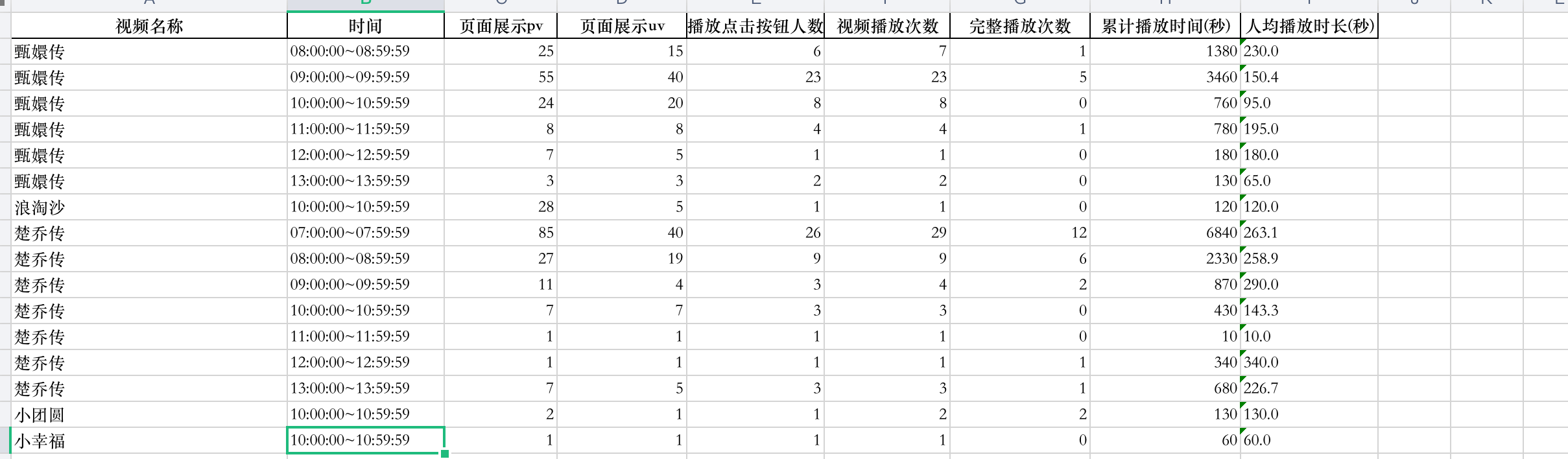处理之后的数据：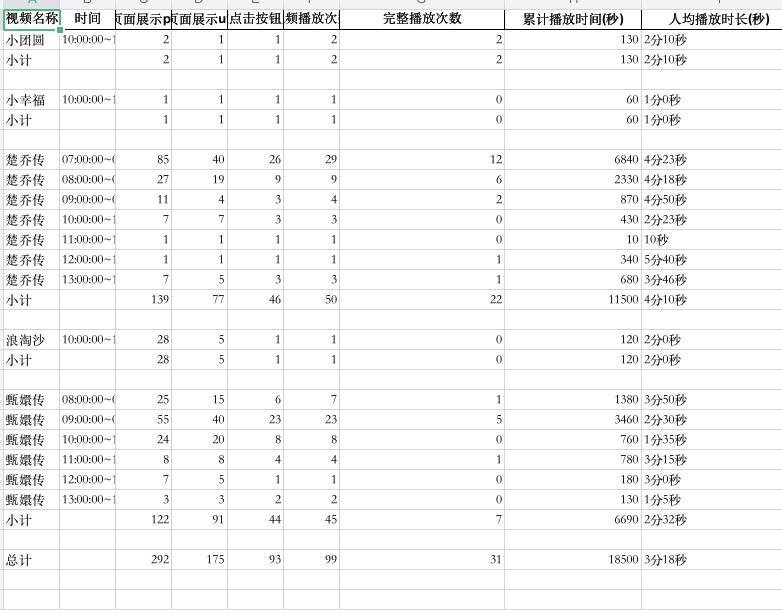展开全文• 如何用pandas同时实现以下分类汇总：合并相同“名称”，“金额”求和，“利率”取均值，日期、类型、主体跟随“名称”合并。 尝试了一些办法，groupby似乎不能同时既求和又求均值&#...python
• 结合pandas.apply函数和轴方向对行列进行统计。 实现 import pandas as pd import matplotlib.pyplot as plt plt.rcParams['font.sans-serif']=['SimHei'] plt.rcParams['axes.unicode_minus']=False #读取数据 ...
需求
现有一批成绩，要求统计每名学生的总成绩并添加“总分”列，统计各科成绩的平均分和标准差并添加相应行。
要点
结合pandas.apply函数和轴方向对行列进行统计。
实现
import pandas as pd
import matplotlib.pyplot as plt

plt.rcParams['font.sans-serif']=['SimHei']
plt.rcParams['axes.unicode_minus']=False

#读取数据
#展示数据
score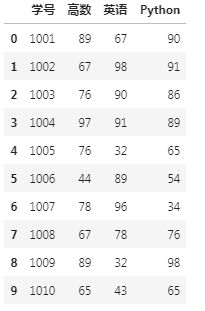#按行求和，添加总分列
score["总分"] = score[['高数','英语','Python']].sum(axis =1)
#score["总分"] = score[['高数','英语','Python']].apply(lambda x:x.sum(),axis =1)
score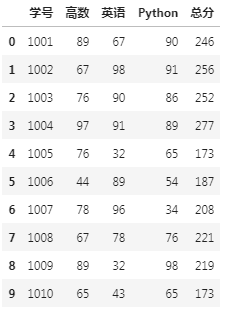score.loc['合计']=score[['高数','英语','Python','总分']].sum(axis=0)
score.loc['平均分']=score[['高数','英语','Python','总分']].mean(axis=0)
score.loc['标准差']=score[['高数','英语','Python','总分']].std(axis=0)
score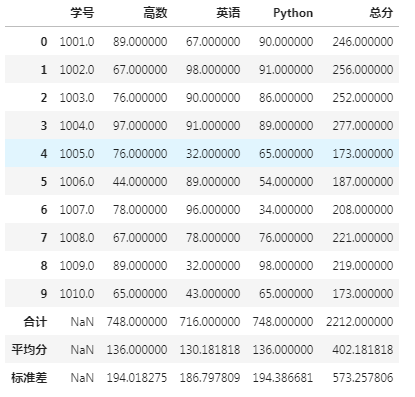展开全文apply axis
• pandas中使用sum()函数，发现返回值的小数位数增加了许多，且max()函数也有类似情况。在python3.6.3中运行以下代码： #求a、b两列的和以及最大值。 m = [{'a':2.4455, "b":3.4444}, {'a':4.4435, &...sum max
• Dataframe汇总计算的主要方法有： Pandas 统计的一些常用方法： frame.idxmax(): 列的最大值 输出每列最大值的索引 np.random.seed(38754) data=np.random.randint(0,15,15).reshape(5,3) frame=...python dataframe
• import pandas as pd from pandas import Series,DataFrame 一、介绍 聚集函数只是将一组值转换为一个标量值，其实还可以将更多类型的函数应用到分组上，下面的transform和apply就提供这样的功能。 二、...apply transform 分组 groupby 函数
• pivot_table(self, values=None, index=None, columns=None, aggfunc=‘mean’, fill_value=None, margins=False, dropna=True, margins_...import pandas as pd #创建数据集 df = pd.DataFrame({"A": ["foo", "fo...
• ## Python分类求和方法

千次阅读 2018-11-27 16:40:51
Python分类求和方法，Excel表格数据为例。 在Excel中经常会有根据列分类求和的需求，如果数据较少,可以在Excel中通过手动筛选的方法，但是数据较多那就比较麻烦啦。 这里需要用到groupby函数，pandas提供了一个...Python excel
• 今天主要记录一下pandas去重复行以及如何分类汇总。以下面的数据帧作为一个例子：  import pandas as pd data=pd.DataFrame({'产品':['A','A','A','A'],'数量':[50,50,30,30]}) pandas判断dataframe是否含有重复...python 去重复
• ## pandas分组统计 - groupby功能

万次阅读 多人点赞 2019-01-05 11:11:16
print("----------将CD作为一组分出来，并计算求和-----------") mapping = {"C":"one", "D":"one"} print(df.groupby(mapping, axis = 1).sum()) print("------------以B分组，求每一组的均值、和、最大值、最小值-...groupby
• ## 【Python】操作Excel之分类汇总

万次阅读 多人点赞 2018-03-31 12:00:21
三月是雷锋月，抓住他的小尾巴，留下一点爱心，分享一下近来几天我学习python操作Excel的体会与技巧：对表格进行分类汇总。 有一个Excel表 （乱序）  我想要操作的就是把所有科目编码为'10101'的'借方金额'与'...Python Excel
• 1、首先导入pandas库，一般都会用到numpy库，所以我们先导入备用： import numpy as np import pandas as pd 2、导入CSV或者xlsx文件： df = pd.DataFrame(pd.read_csv(‘name.csv’,header=1)) df = pd.DataFrame...python
• 但是感觉用excel自身的功能感觉操作并不简单，于是决定使用pandas解决，并尽可能多的了解一下pandas中的groupby 函数 （1）代码解决 # 第一个参数：哪个工作薄，第二个参数，哪个工作表 >>> df = pd.read_...python excel 数据分析
• 它们大部分都属于约简和汇总 统计，用于从Series中提取单个值(如sum或mean)或从DataFrame的行或 列中提取一个Series。跟对应的NumPy数组方法相比，它们都是基于没有缺 失数据的假设而构建的。看一个简单的DataFrame:...Python数据分析 统计计算
• python pandas 操作集锦 ** 载入数据集 df = pd.DataFrame({ '学生姓名':['小红','小花','小明','小军','小雷'], '性别':['女','女','男','男','男'], '语文成绩':[58,78,96,64,85], '数学成绩':[90,69,45,35,57...python 数据分析 机器学习 AI
• 按照 商品-价格 分类汇总，这里调用 reset_index() 方法，让结果看起来更直接一些。 生成excel: df_result . to_excel ( 'E:/dat/myfile/分类统计各类型商品销售额.xlsx' ) 第四步 合并两列单元格 ...excel合并单元格
• 主要用到的是pd.Grouper方法 ...import pandas as pd from datetime import datetime from dateutil.parser import parse df_A = pd.DataFrame([['2019-10-26', 300], ['2019-10-27', 500], ...dataframe
• Pandas 对象拥有一组常用的数学和统计方法，他们大部分都属于约简和汇总统计，用于从 Series 中提取单个值（如 sum/mean），或者从 DataFrame 的行或列中提取一个 Series。 In : df = DataFrame([[1,np.nan],...
• part4 多表联合查询(join)1、多表联合excel中是用vlookup，pandas中是用merge和join，数据库中用join。#读取第一张表 如果，没有on='ID'这一参数，也能得出结果，因为merge能自动去在两张表查出相同的列名。但如果...
• 这是因为groupby在计算后行数会仅仅保留groupby的分类行数。 没有办法，只能用中间表+merge的方法添加新列，无形中代码量和内存占有率都高了很多。 dat_input_equip = dat.groupby(by=['step','equip_id'])['glass_...
• 对于pandas中的Dataframe,如果需要按照列A进行分组，将同一组的列B求和,可以通过下述操作完成：df = df.groupby(by=['column_A'])['column_B'].sum()　生成的数据类型是Series,如果进一步需要将其转换为dataframe,...python
• # 参考本人博文：pandas统计学-相关性系数（pearson、spearman、kendall，pointbiserialr） s.corr(s1) #0.5508422083307387 Series实例 2：- unique唯一值 pd.Series([2,1,3,3,2,1], name='A').unique()#...
• 数据分类处理： 分组：先把数据分为几组 用函数处理：为不同组的数据应用不同的函数以转换数据 合并：把不同组得到的结果合并起来 数据分类处理的核心： groupby()函数 6.高级数据聚合 （1） 可以使用pd.merge()...
• Pandas中通过.sort和.loc函数也可以实现这两 个功能。.sort函数可以实现对数据表的排序操作，.loc函数可以实现对数据表的筛选操作。本篇文章将介绍如果通过Pandas的这两个函数完成Excel中的筛选和排序操作。 ...
• import pandas as pd app = xw.App(visible=False,add_book=False) file_path = r'D:\python_file\销售表' file_list = os.listdir(file_path) # 列出文件夹下所有文件和子文件夹的名称 for i in file_list: if os.......